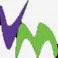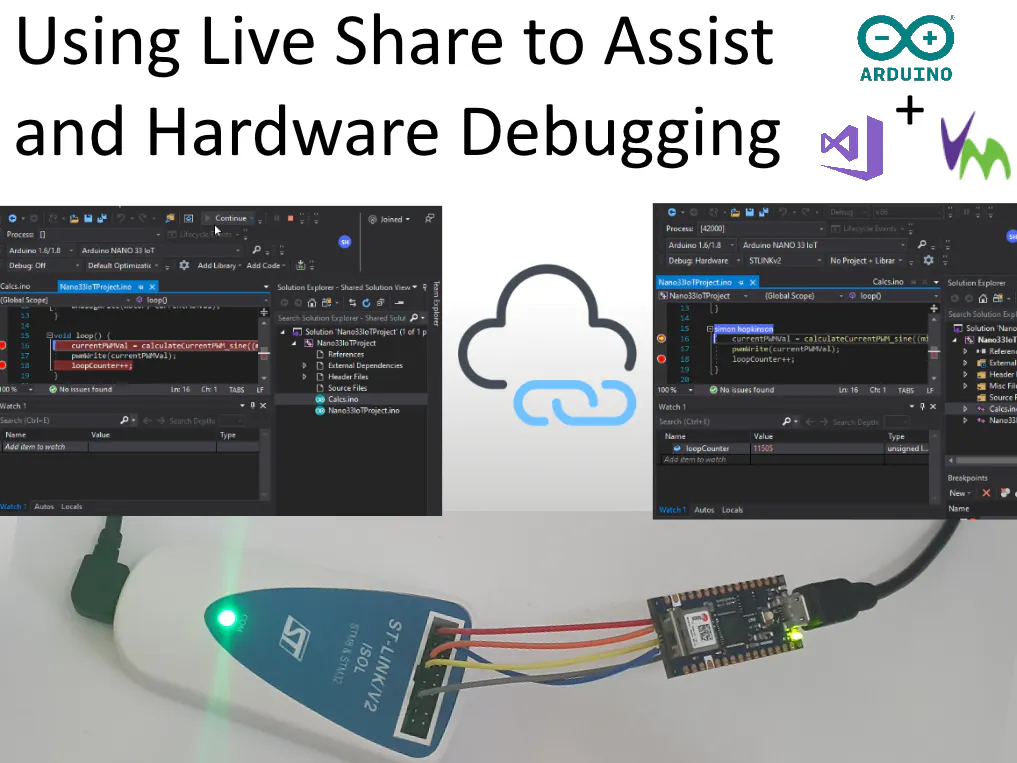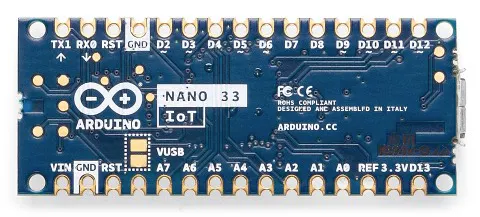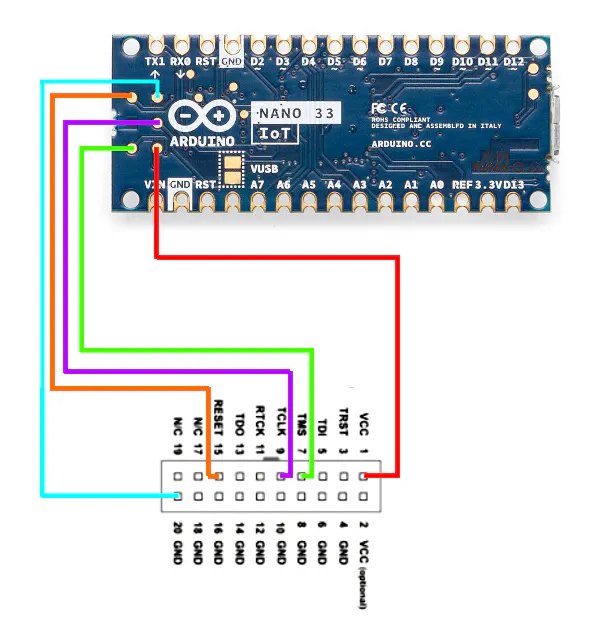# Arduino Nano 33 IoT Remote Debugging + Coding

Working through a problem, but only you have the hardware? Want to work with someone remotely as if they were there? This is for you...

IntermediateFull instructions provided4## Things used in this project

### Hardware componentsArduino Nano 33 IoT Or any Other supported board
×1
 STMicroelectronics STLinkv2 Hardware Debugger Or any Other supported Hardware Debugger
×1Jumper wires (generic)
×1

### Software apps and online servicesArduino IDEMicrosoft Visual Studio 2017
 Visual Micro

## Schematics

### Example Nano33 IoT Connection Diagram

How to Connect the Nano-33 IoT to a Hardware Debugger## Code

### NanoDebugExample.ino

Arduino
Basic Sketch to use with debugging, use with other Ino file to use the same code as shown in the example.
```const int motor = 12;
int currentMotorPWM = 0;
unsigned long currentPeriodStartTime = 0;
unsigned int currentPeriod_MS = 1000;
unsigned int currentResolution = 254;
unsigned int maxResolution = 254;
int currentPWMVal = 0;
unsigned long loopCounter = 0;

void setup() {
pinMode(motor, OUTPUT);
analogWrite(motor, currentPWMVal);
}

void loop() {
currentPWMVal = calculateCurrentPWM_sine((millis() - currentPeriodStartTime) % currentPeriod_MS);
pwmWrite(currentPWMVal);
loopCounter++;
}
```

### Calcs.ino

Arduino
Additional Code to be used in Debugging Sketch Example
```// Encapsualtion
int calculateCurrentPWM_spiky(unsigned long periodTime) {
return calculateCurrentPWMMultiplier(periodTime) * currentResolution;
}

int calculateCurrentPWM_sine(unsigned long periodTime) {
return
round(
abs(int(
(
sin(
(
double(calculateCurrentPWM_spiky(periodTime))
/ 165.00
)
)
)
* double(currentResolution)
)
)
);
}

int oldValue = -1;
void pwmWrite(int value) {
if (oldValue != value) {
analogWrite(motor, value);
oldValue = value;
Serial.print(",");
Serial.print(value);
}
}

double calculateCurrentPWMMultiplier(unsigned long periodTime) {
if ((double(periodTime % currentPeriod_MS) / double((currentPeriod_MS / 2.00))) <= 1.00) {
return (double(periodTime % currentPeriod_MS) / double((currentPeriod_MS / 2.00)));
}
else {
return (1.00 - ((double(periodTime % currentPeriod_MS) - double((currentPeriod_MS / 2.00))) / double((currentPeriod_MS / 2.00))));
}
}
```

## Credits

### visualmicro

2 projects • 4 followers